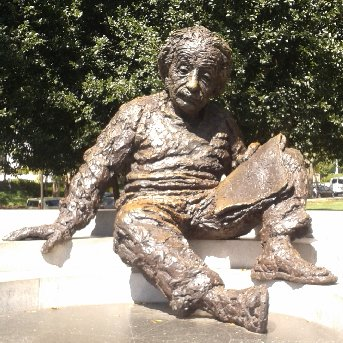# Where it all began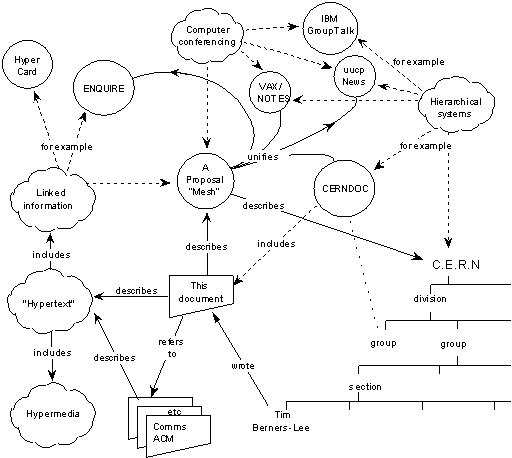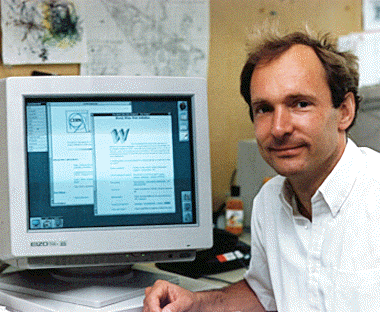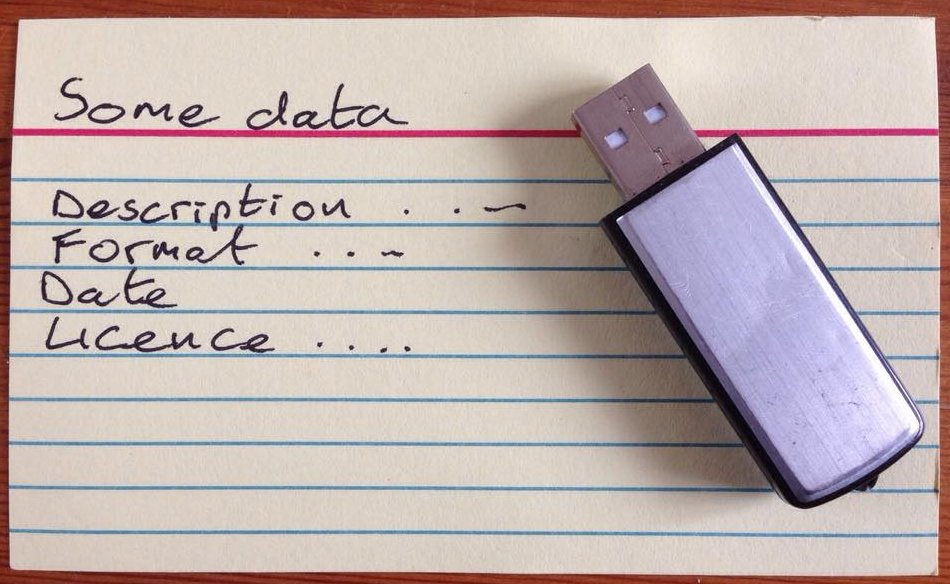Photo: Rosie Sutton

# The Web of Data

Linked Data uses the concepts and technologies of the Web to describe the world

Here is a picture.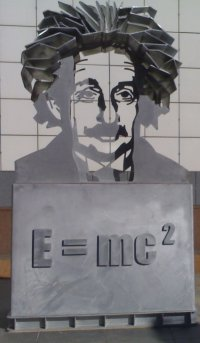Here is a picture.

Of a sculture.Here is a picture.

Of a sculture.

Of Albert EinsteinHere is a picture.

Of a sculture.

Of Albert Einstein

And his famous equation: e=mc2

Here is a http://en.wikipedia.org/wiki/Image

And his famous equation: http://en.wikipedia.org/wiki/Mass-energy_equivalence